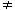# Aptitude - Logarithm - Discussion

### Discussion :: Logarithm - General Questions (Q.No.1)

1.

Which of the following statements is not correct?

 [A]. log10 10 = 1 [B]. log (2 + 3) = log (2 x 3) [C]. log10 1 = 0 [D]. log (1 + 2 + 3) = log 1 + log 2 + log 3

Explanation:

(a) Since loga a = 1, so log10 10 = 1.

(b) log (2 + 3) = log 5 and log (2 x 3) = log 6 = log 2 + log 3log (2 + 3)log (2 x 3)

(c) Since loga 1 = 0, so log10 1 = 0.

(d) log (1 + 2 + 3) = log 6 = log (1 x 2 x 3) = log 1 + log 2 + log 3.

So, (b) is incorrect.

 K.Manohar Reddy said: (May 22, 2011) Its absolutely correct.

 Begum said: (Aug 21, 2011) Its great.

 Venu said: (Oct 2, 2014) If they say log 6 = log 2 + log 3, then log (2+3) = log 2 + log 3. Can someone explain ?

 Vikash said: (Oct 20, 2014) log 6 = log(2*3) = log 2 + log 3. But log(2+3) =log 5 which is not equal to log 6. So, log(2+3) != log 2 + log 3.

 Tendai said: (Jan 18, 2015) What about 243 base 9?

 Akshayaa said: (Mar 19, 2015) What about 828 with base 2?

 Murali said: (Jul 6, 2015) 243 base 9. This mainly 243 = 3*3*3*3*3 = 3^5. And 9 = 3*3 = 3^2. So answer is 5/2. I dot no how to write log in keyboard.

 Palak Kapoor said: (Oct 1, 2015) If a=5 and b=4 then what is the value of log (a-b) (a^2-b^2-2^b)?

 Shashi said: (Oct 4, 2015) Its awesome.

 Ssd said: (Oct 13, 2015) What is log to the base 5 512?

 Garvit said: (Jan 18, 2016) Option D can't be considered answer. It's tricky actually: LHS: log (1+2+3) = log 6. [1+2+3 = 6]. RHS: log1 + log2 + log3 = log (1x2x3) = log 6. Since LHS = RHS (no matter how it comes) D isn't the option.

 Suhaas said: (May 1, 2016) @Palak Kapoor. The answer is undefined. log{5 - 4}[5^2 - 4^2 - 4] log{1}[25 - 16 - 16] log[25 - 32] log[- 7] log value for -ve is undefined.

 Sylvester said: (Jun 13, 2016) The given answer is absolutely correct.

 Meenakshi said: (Jul 19, 2016) The anwer is absolutely correct according to log properties. It's log a + log b = log(a * b). not log(a + b) = log(ab).

 Ravi said: (Dec 3, 2016) @all. B is absolutely correct.

 Disha Jhan said: (Mar 11, 2017) The answer B is correct. I agree with it.

 Isaac said: (Mar 14, 2017) Can someone help me understand how D is a true statement? If log6=10^6=1,000,000 how is that equal to log1+log2+log3? =10^1+10^2+10^3, =10+100+1000, =1110.

 Sanyal said: (Mar 14, 2017) Correct, Thanks for the given explanation.

 Dhaval said: (Nov 23, 2017) D is also incorrect. log(1+2+3) = log 1* log 2 * log 3.

 Riya Raj said: (Mar 15, 2018) I'm also confused because it both the cases results are coming the same soo however it's different. Please explain me.

 Deepak said: (May 6, 2018) I am not understanding this. Can anyone explain by taking general variable?

 Kity said: (Jun 14, 2018) How, Please explain this.

 Abhishek said: (Aug 6, 2018) The answer B is correct. I agree with it.

 Omkar Rajale said: (Aug 24, 2018) Please explain the option D.

 Keerthana said: (Jan 31, 2019) Thanks all for explaining.

 Shivam Ghorpade said: (Jun 30, 2019) Thanks all for explaining.

 Sneha said: (Apr 29, 2020) Thanks for the explanation.

 Girmay Yohanness said: (May 6, 2020) Thanks for the explanation.

 Swaroop said: (Jul 1, 2020) According to me, Option B and D, both are incorrect.

 Swaroop said: (Jul 1, 2020) Answer D is correct too, since. log(1+2+3)=log(6)=log(1*2*3)=log1+log2+log3.